Binary Tree is the non-linear and dynamic data structure. Binary tree can have at most 2 children i.e tree can have 0,1,or 2 nodes in tree.

The main  difference between Tree and Binary tree Tree can have infinite number of children but binary tree can have at most 2 children. Understanding Tree Concepts

Diagrams given below showing different forms of BT.

[A] has only one node that is root of the tree.

[B] Root has only one left child.

[C] has one right and again right node has one right child.

[D] has proper two children one left and one right child.

All trees are the form of binary tree, there is only one condition for Binary Tree that tree can not have more than 2 children.Types of binary tree. ByTechAchievers.com

Types Of Binary Tree -

1. Strict or Proper Binary Tree -

A tree that can have either 2 or 0 children called strict binary tree.

The difference between binary tree and strict binary tree , you can see that binary tree can have at most 2 (0,1,2) but with strict binary tree it can not have 1 child, it can have either 2 or 0 children.

From the given example you can see that,

[A] has node number 2 that has 1 child it does not fulfill strict binary tree condition.

[B] fulfills the condition "each node can have either 2 or 0 children", so that it is strict binary tree.Strict or Proper binary tree. ByTechAchievers.com

2. Complete Binary Tree -

For Complete binary tree there are two conditions has to be filled.

A)
Each level should be fulfilled except last level i.e levels will be filled
one by one.First level will be fulfilled first then next level can have  children.

B) Every parent will have left child entry first after that right child will take

place

so that after 1st condition at last level left position of
the node should not be  vacant.

According to diagram we can understand different types of complete trees examples.Complete binary tree. ByTechAchievers.com

[A] At level 2 node does not have right child node so that it doesn't fulfilled the level.

[B] At level 2 number of nodes are correct but node 4 should have child entry first. Left position of node should not be vacant.

[C] C is the complete binary tree example. It fulfills both conditions.

3. Perfect Binary Tree -

As the name suggesting perfect means a tree that all levels are fulfilled.

A tree that each node should have two children and all leaves should be in same level known as perfect binary tree.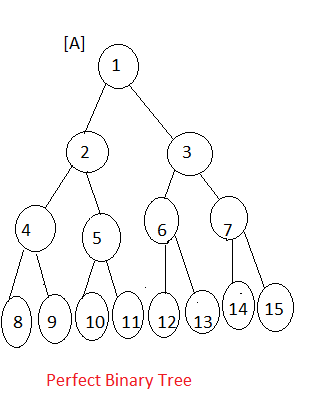Perfect binary tree. ByTechAchievers.com

Diagram shows the example of perfect binary tree, all levels are fulfilled

and all leaves are at same level.

3. Balanced Binary Tree -

Balanced binary tree means the left sub-tree and right sub-tree heights difference should not be greater then 1.

How we can get height of the tree = number of edges from in a longest path from root to leaf.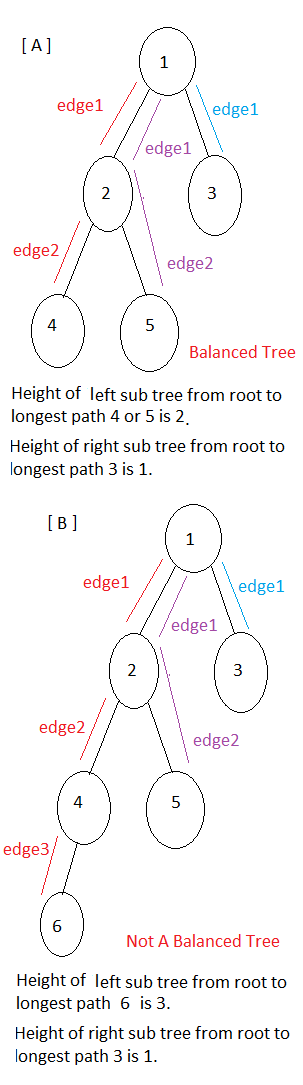Balanced binary tree. ByTechAchievers.com

As we can understand with balanced tree example,

[A] Is balanced tree because the left and right sub trees height difference is 1 which satisfy the balanced tree condition.

[B] Is not a balanced tree because the left and right sub trees height difference is 2 which does not satisfy the balanced tree condition.

Understanding/Calculating Number Of Nodes In A Tree -

Here we will learn how to find number of nodes in binary tree.If we assume that L is level of the tree then each level can have maximum 2L-1 nodes.

 Level 2L-1 Maximum Number of Nodes In each Level 1 21-1  = 20 1 2 22-1  = 21 2 3 23-1  = 22 4 4 24-1  = 23 8  and so on...

As we know that number of edges (or branches you can say ) in the longest path from root to leaf node is called height.

If we calculate number of nodes with height of the tree,
``````

20 + 21 + 22 + 23 + . . . . . + 2H     { H - Height of the tree }

= 2H+1 - 1   { H+1 is the number of levels }

= 2L - 1

Let's calculate number of nodes in a perfect tree
example: number of levels are 4 so that number of nodes in a tree

= 24 - 1

= 16 -1 = 15

``````
Operations Of Binary Search Tree -

Let's understand first the difference between Binary Tree and Binary Search Tree (BST).

Binary tree that can have at most 2 children, whereas Binary Search Tree is the sorted binary tree, in BST all elements that are less then root will be at left side and elements that are greater than root will be at right side.

Let's talk about node structure of BST, each node in tree will have will have data value of the node, reference of its left child and reference of it's right childIf there is no child available then node reference would be null.Binary Tree Node Structure. ByTechAchievers.com

1.
Define class for node.
``````
class BTreeNode {

BSTNode left;

BSTNode rigth;

String dataValue;

}
``````

2.
Creating new node, initially each node will have null reference for left and
right child.
``````
BSTNode new_node = new BSTNode ();

new_node.dataValue = x;

new_node.left = Null;

new_node.right = Null;
``````

3.
Insertion process in BST

Initially root of the node is null, check if root is null then new node would be the root of tree. I will maintain currentNode here, initially it will have root value but during the process its value will be updated.
``````
If (root == null ) {

root = node;

}

currentNode = root; ``` ```
Check if element (that has to be inserted) < currentNode (initially its  a root), again check if currentNode -> left node is null, create
new node as left child otherwise currentNode would be left child of root.
``````
if (element < currentNode.dataValue) {

if (currentNode.left == null) {

currentNode.left = createNode

}

else {

currentNode = currentNode.left;

}

``````
Check if element (that has to be inserted) > currentNode (initially its a root), again check if currentNode -> right node is null, create
new node as right child otherwise currentNode would be right child of root.
``````
if (element > currentNode.dataValue) {

if (currentNode.right == null) {

currentNoderight = createNode

}

else {

currentNode = currentNode.right;

}
``````

4.
Traversal process in BST

Traversing of the tree that is go through with all the nodes.

As we know that tree nodes are arranged in hierarchical form so that root node comes first, all less nodes or children are arranged
in left of the root and greater nodes or children are arranged in right.

We can traverse tree in different ways like as per our requirement,

Inorder Traversing -

Reading all left nodes first

Visit root node of the tree

Reading all right nodes.Inorder Traversing. ByTechAchievers.com

Let's understand by flow no. - 1 ,2 and 3

1 - Left - Root - Right = 4 2 7

2 - Left - Root - Right = (left is done by 1st flow) 10  (read right sub-tree see flow 3)

3 - Left - Root - Right = (left of right sub-tree) 12 23 45

Inorder Traversing = 4 2 7 10 12 23 45

Preorder Traversing -

Visit root node of the tree

Reading all left nodes first

Reading all right nodes.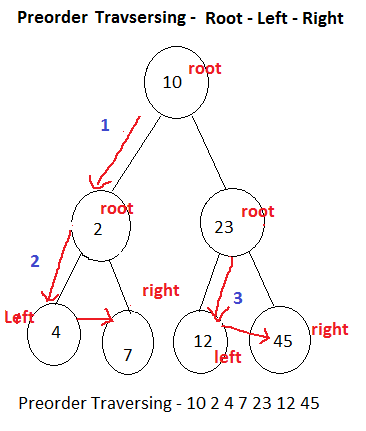Preorder Traversing.  ByTechAchievers.com

Let's understand by flow no. - 1 ,2 and 3

1 - Root - Left - Right = 10 (read left sub-tree - see flow 2).

2 - Root - Left - Right = 2 4 7

3 - Root - Left - Right = (right sub-tree) 23 12 45

Preorder Traversing = 10 2 4 7 23 12 45

Postorder Traversing -

Reading all left nodes first

Reading all right nodes.

Visit root node of the treePostorder Traversing. ByTechAchivers.com

Let's understand by flow no. -
1 ,2 and 3

1 - Left - Right - Root = 4 7 2 (start from left sub-tree).

2 - Left - Right - Root = 12 45 23 (same order for right sub-tree)

3 - Left - Right - Root = (left and right sub-trees are done by 1 & 2) 10

Postorder Traversing = 4 7 2 12 45 23 10

4.
Deletion process in BST

Deleting node from tree is same as we did with linked list, remove the link of that node from tree. Node will be deleted.

While deleting node first search the node has to be deleted in BST.

If node value is less then root then search in left sub-tree otherwise search in right sub-tree.

If node has no left and no right child i.e. it is a leaf node remove it's reference from it's parent node. If leaf node is the left child of parent node then set parent - > left = null, if  leaf node is the right child of parent node then set parent - > right = null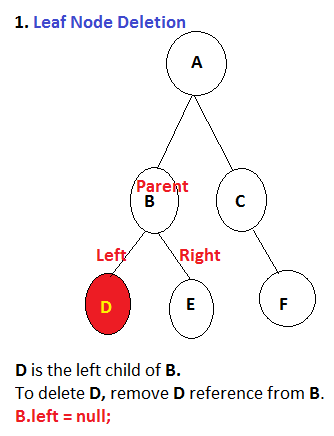Leaf-Node Deletion In BST. ByTechAchievers.com

If node has only one child. If we have to remove a node that has only left child then left child would become the parent child.

parent = parent - > left. If parent has right child then right child would become parent node parent = parent - > rightParent reference would be removed from BST.One Child Deletion In BST. ByTechAchievers.com

If node has two children. In this case node has both left sub-tree and  right sub-tree, we have to replace node (has to be deleted) with either maximum node value from left sub-tree or minimum node value from right sub-tree.

In my Java code example I have replaced node with "minimum value" from right sub-tree.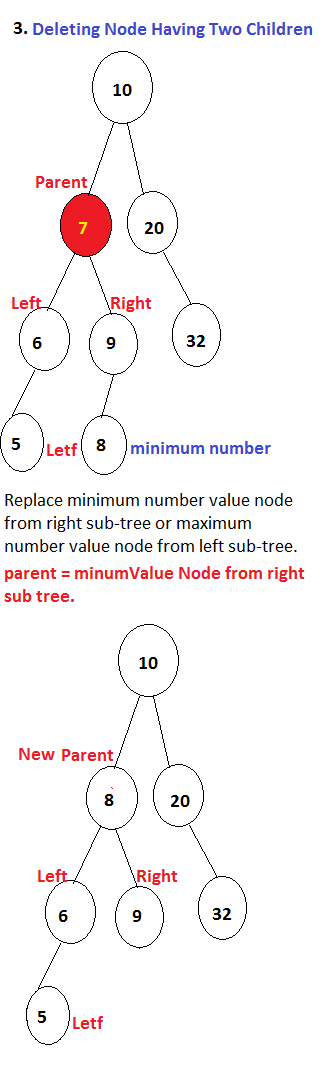Two Children Node Deletion In BST. ByTechAchievers.com

Implementation Of Binary Search Tree In Java -
``````
class BSTNode {

BSTNode left;

BSTNode right;

int value;

BSTNode (int element) {

this.left = null;

this.right = null;

this.value = element;

}

} // end of the BSTNode class

public class BSTOperationExample {

private BSTNode root;

public static void main (String args[]) {

BSTOperationExample bst = new BSTOperationExample();

String dataList[] ={"5","2","4","7","9","1","90","23","10"};

for (int i=0 ; i <dataList.length ; i++) {

// calling method to insert element in BST

bst.insertBSTNode(Integer.parseInt(dataList[i]));

}

//Traversing of nodes in inOrder

System.out.print("Inorder Traversing -  ");

bst.inOrderTraversing(bst.getRoot());

System.out.println();

//Traversing of nodes in preOrder

System.out.print("Preorder Traversing -  ");

bst.preOrderTraversing(bst.getRoot());

System.out.println();

//Traversing of nodes in postOrder

System.out.print("Postorder Traversing -  ");

bst.postOrderTraversing(bst.getRoot());

//Deleting node from BST

System.out.println("\n\nDeleting element 10 from BST -  ");

bst.deleteBSTNode(bst.getRoot(),10);

//Traversing of nodes in inOrder

System.out.println("\nInorder Traversing After Deleting Node -  ");

bst.inOrderTraversing(bst.getRoot());

} //End of the main

//Setter getter for root

public BSTNode getRoot(){

return root;

}

public void setRoot(BSTNode root){

this.root = root;

}

//Method to insert element in BST

public void insertBSTNode (int element) {

if (root == null ) {

//Create root node

root = new BSTNode(element);

//Maintaining root

setRoot(root);

}

insertChildrenNode(getRoot(),element);

} //End of the insertBSTNode

// Method to insert children node or root

public BSTNode insertChildrenNode (BSTNode root ,int element) {

BSTNode currentNode = null;

// Root element checking

if (element == root.value) {

return root;

}

//Left child condition

if ( element < root.value ) {

if (root.left == null) {

//Create left child

root.left = new BSTNode (element);

return root.left;

}

else {

/*If left is available, make it current node

will send it for further checking */

currentNode = root.left;

}

}

//Right child condition

if ( element > root.value ) {

if (root.right == null) {

//Create right child

root.right = new BSTNode (element);

return root.right;

}

else {

/*If right is available, make it current node

will send it for further checking */

currentNode = root.right;

}

}

return  insertChildrenNode(currentNode,element);

}//End of the insertChildrenNode

// Method to traverse BST in inOrder

public void inOrderTraversing ( BSTNode root ) {

if ( root != null ) {

//Left node traversing

inOrderTraversing (root.left);

//Visit root value

System.out.print(root.value + "  ");

//Right node traversing

inOrderTraversing (root.right);

}

}//End of inOrderTraversing

// Method to traverse BST in preOrder

public void preOrderTraversing ( BSTNode root ) {

if ( root != null ) {

//Visit root node

System.out.print(root.value + "  ");

//Left node traversing

preOrderTraversing (root.left);

//Right node traversing

preOrderTraversing (root.right);

}

}//End of preOrderTraversing

// Method to traverse BST in postOrder

public void postOrderTraversing ( BSTNode root ) {

if ( root != null ) {

//Left node traversing

postOrderTraversing (root.left);

//Right node traversing

postOrderTraversing (root.right);

//Visit root node

System.out.print(root.value + "  ");

}

}//End of postOrderTraversing

// Method to delete node from BST

public BSTNode deleteBSTNode (BSTNode root, int element) {

if(root != null) {

if (element < root.value) {

root.left = deleteBSTNode (root.left,element);

}

else if ( element > root.value) {

root.right = deleteBSTNode (root.right,element);

}

else {

//Deleting leaf node

if (root.left == null && root.right == null) {

System.out.println(element+ " has been deleted");

return null;

}

//Deleting 1 child node

else if (root.right == null ) {

System.out.println(element+ " has been deleted");

return root.left;

}

else if (root.left == null ) {

System.out.println(element+ " has been deleted");

return root.right;

}

//deleting two  children node

else {

//Search minimum number from right sub tree

int minimum_number = getMinimum(root.right);

//Replace minimum number with deleting number

root.value = minimum_number;

System.out.println(element+ " has been deleted");

return root.right;

}

}

}

return root;

} //End of deleteBSTNode

//Method to find minimum number from tree

public int getMinimum (BSTNode node) {

//Read left child to get minimum number

if(node.left !=null) {

getMinimum(node.left);

}

return node.value;

}//End of getMinimum

}//End of the class

``````

OUTPUT -

Inorder Traversing -  1  2  4  5  7  9  10  23  90
Preorder Traversing -  5  2  1  4  7  9  90  23  10
Postorder Traversing -  1  4  2  10  23  90  9  7  5

Deleting element 10 from BST -
10 has been deleted

Inorder Traversing After Deleting Node -
1  2  4  5  7  9  23  90

Thanks for reading, please do share and comment your suggestions.